StatLect

# Prior probability

The prior probability is the probability assigned to an event before the arrival of some information that makes it necessary to revise the assigned probability.

The revision of the prior is carried out using Bayes' rule.

The new probability assigned to the event after the revision is called posterior probability.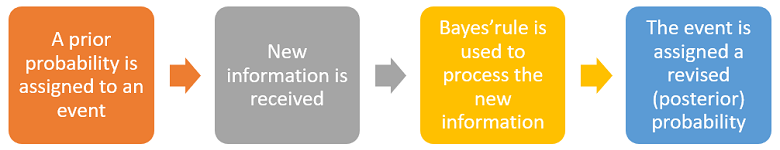## Bayes' rule

The arrival of new information is processed using Bayes' rule: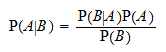The prior probability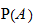is assigned to the eventbefore receiving the information that the eventhas happened.

After receiving this information, the prior probability is updated and the posterior probability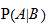is computed.

In order to perform the calculation, the conditional probability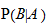needs to be known.

## Example

Suppose that an individual is extracted at random from a population consisting of two ethnic groups, ABC and XYZ.

We know that:

• 30% of individuals belonging to group ABC have incomes below the poverty line;

• the corresponding proportion for the population as a whole is 20%;

• 40% of the population is made of individuals belonging to group ABC.

If we extract an individual whose income is below the poverty line, what is the probability that she belongs to group ABC?

This conditional probability can be computed with Bayes' rule.

The quantities involved in the computation are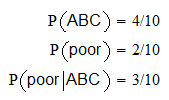The prior probability is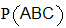, the probability of belonging to group ABC.

The posterior probability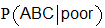can be computed thanks to Bayes' rule: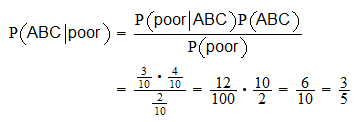## Priors in Bayesian statistics

Prior probability is a fundamental concept in Bayesian statistics.

In Bayesian inference, the following conceptual framework is used to analyze observed data and make inferences:

• there are several probability distributions that could have generated the data;

• each possible distribution is assigned a prior probability that reflects the statistician's subjective beliefs and knowledge accumulated before observing the data;

• the observed data is used to update the prior, by using Bayes' rule;

• as a result of the update, all the possible data-generating distributions are assigned posterior probabilities.

## Prior distribution

In Bayesian statistics, it often happens that the set of probability distributions that could have generated the data is indexed by a parameter.

The parameter is seen as a random variable and it is assigned a subjective probability distribution, which is called the prior distribution.

The prior distribution is then updated, using the observed data, and a posterior distribution is obtained.

Example Suppose that we observe a number of independent realizations of a Bernoulli random variable (i.e., a variable that is equal to 1 if a certain experiment succeeds and 0 otherwise). In this case, the set of data-generating distributions is the set of all Bernoulli distributions, which are indexed by a single parameter (the probability of success of the experiment). The distribution assigned to the parameter before observing the outcomes of the experiments is the prior distribution (usually a Beta distribution).

## Examples of priors in Bayesian statistics

To better understand the meaning of a prior in Bayesian statistics, you can read the following examples:

## Unconditional probability

The prior probability is also often called unconditional probability, as opposed to the posterior probability, which is a conditional probability.

## More details

You can find a more exhaustive explanation of the concept of prior probability in the lecture entitled Bayes' rule.

Previous entry: Precision matrix

Next entry: Probability density function